## SERVO MOTOR CONTROL USING PIC16F877ASERVO MOTOR
Servo motors are used to control many things as it offers very precise rotation of 1 degree. For this it uses the feedback from a potentiometer connected.

For controlling the angle it require a pulse of certain duty cycle which can be achieved using the pulse with modulation (PWM) signal.
1. For 0 degree it takes a 800us pulse (us = microseconds)
2. For 90 degree it takes a 1500us pulse
3. For 180 degree it takes a 2200us pulse
Note:- This pulse can vary with different model of servo motor.

### We can control is using the PIC16F877A

For this we have to understand the PWM working of the micro-controller.

PIC has overall 3 timers
• TIMER0
• TIMER1
• TIMER2

We will use the TIMER1 for generating the PWMRegister Associated with TIMER1 as a TIMER/COUNTER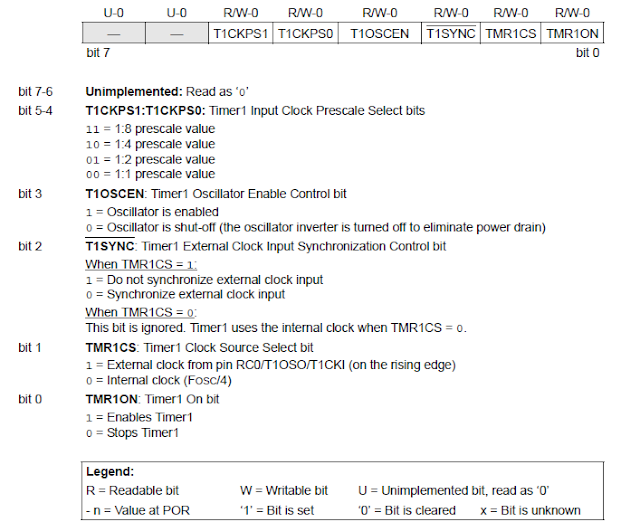TIMER1 Control RegisterTIMER1 Block Diagram The prescaler value is set to 1:1 The Crystal frequency used is 20MHz You can connect the Bluetooth and send the command to control the angle of the servo motor. If the data received is 0 the motor rotates to 0 degree If the data received is 1 the motor rotates to 90 degree If the data received is 2 the motor rotates to 180 degree Note:- Compiler or the software used here is MikroC pro for PIC THE CODE: -
/*code for generating 800us, 1500us and 2200us pulse for controlling servo motor 0, 90 and 180 degree
 Send the code from the bluetooth to the PIC16F877A for conrolling the angles   Crystal oscillator connected is 20MHz   Author:- ASHISH KUMAR       */ char a;             //char for recieving data from bluetooth unsigned lval=0x60; unsigned hval=0xF0; void interrupt(){   if(TMR1IF_bit){      PORTB.F0=!PORTB.F0;      TMR1L=lval;      TMR1H=hval;      TMR1IF_bit=0;   }   if(PIR1.F5)  {     while(PIR1.F5==0);     a=RCREG;     if(a=='0'){       //for 800us pulse (0 degree)       lval=0x60;       hval=0xF0;     }     else if(a=='1'){  //for 1500us pulse (90 degree)       lval=0xB4;       hval=0xE2;     }     else if(a=='2'){      //for 2200us pulse (180 degree)       lval=0x08;       hval=0xD5;     }   } } void main(){   OPTION_REG= 0x87;   TRISB.F0=0x00;   T1CON.F5=0;   T1CON.F4=0;   TMR1L=lval;   TMR1H=hval;   TMR1IE_bit=1;   INTCON.GIE=1;   INTCON.PEIE=1;   TMR1ON_bit=1;   TRISC.F6=0;   TRISC.F7=1;   SPBRG=32;   TXSTA=0x20;   RCSTA=0x90;   PIE1.F5=1;   PIE1.F4=0;   while(1){     //write your code   } } SIMULATION OUTPUT: -Proteus SimulationsPulse Output800us Pulse1500us Pulse2200us Pulse

## LINE FOLLOWING ROBOTLINE SEGUIDOR

### Components Required: -

1. Arduino Uno or any other micro-controller
2. IR sensors or module
3. L293D or module
4. DC motor with wheels
5. Frame
6. Connecting wires
7. LED's

### Connection Diagram of IR sensorsIR transmitter and receiver connections

In the figure the IR0, IR1, IR2, IR3 and IR4 represents the transmitter and D0, D1, D2, D3 and D4 represents the receiver.
The receiver IR is connected to the analog pins of Arduino uno or any other micro-controller.

Note: - Adjust the placing of the IR sensor after measuring the distance and place them in this order and way only. Further if you want to change the positioning then you should make slight adjustment in the code accordingly.

## MOTOR DRIVER IC L293DL293D IC

This IC can controll two DC motor. And can be used to control left and right motor in line following robot.PIN DIAGRAM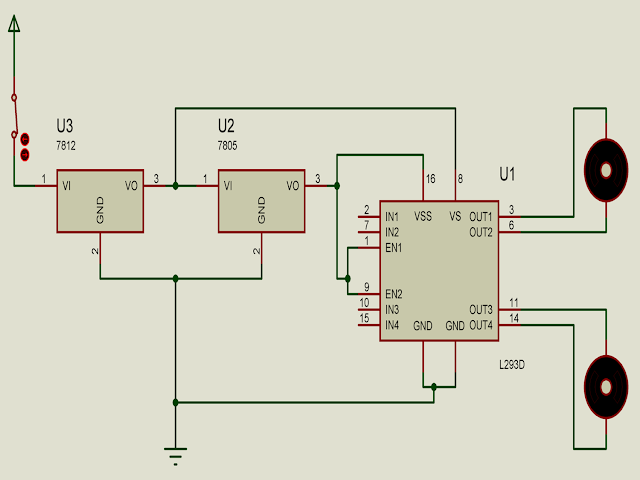BASIC CONNECTION DIAGRAMPIN CONNECTIONS FOR ARDUINO

Note: - After reading the code find the connection of indicator and connect the indicator according to your need.

## Friday, 23 June 2017

### IC CD4511 for controlling Common Cathode Seven Segmented Display

Link for the PDF :- IC CD4511B pdf

1.   PIN DESCRIPTION: -
§  Operating Voltage 2-V to 6-V Vcc
§  10mA at 6V

Four address inputs (A, B, C, D), an active-low blanking (BL) input, lamp-test (LT) input, and a latch-enable (LE) input that, when high, enables the latches to store the BCD inputs. When LE is low, the latches are disabled, making the outputs transparent to the BCD inputs.
Note: -It is used to control Common Cathode Seven Segmented display.

2.  FUNCTION DIAGRAM: -

3.  FUNCTION TABLE: -

## Link for the PDF :- IC CD4511B pdf

4.  LOGIC DIAGRAM: -

5.  CIRCUIT DIAGRAM: -

Seven Segment Display PIN OUT

## Sunday, 18 June 2017

### Three methods of finding Anode and Cathode of the LED (Light Emitting Diode)

Q) WHAT IS LED?????????
A light-emitting diode (LED) is a two-lead semiconductor light source. It is a p–n junction diode that emits light when activated.When a suitable voltage is applied to the leads, electrons are able to recombine with electron holes within the device, releasing energy in the form of photons. This effect is called electroluminescence, and the colour of the light (corresponding to the energy of the photon) is determined by the energy band gap of the semiconductor. LED's are typically small (less than 1 mm^2 ) and integrated optical components may be used to shape the radiation pattern.

HOW DOES LED LOOKS LIKE?Different Types of LED's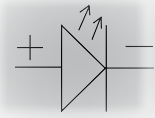Symbol of LED

HOW TO FIND POSITIVE (ANODE) AND NEGATIVE (CATHODE) TERMINAL...........

It's quiet easy............
There are three methods:-

## FIRST METHOD

By looking at there legs we can figure out the anode and cathode.
The Leg are of different size,  i.e., The shorter leg is the NEGATIVE or CATHODE of the LED and the longer one is the POSITIVE or ANODE.
So simple.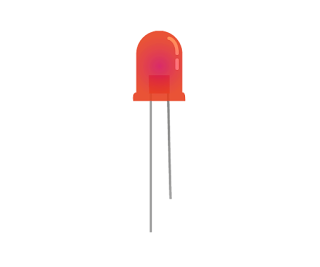A Normal LED
This LED has legs of different length but there is a possibility that you get the LED with both legs of same length.LED with both legs of same length

Now what to do, here comes the

## SECOND METHOD

By looking inside the LED.
You can look inside the LED and find the ANVIL and POST.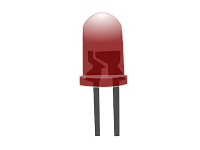In this figure you can clearly see two structure of different width. The one with the greater width is the ANVIL and other is POST

So now clearly the Anvil that has the greater width and is NEGATIVE or CATHODE where as the Post has the smaller width and is POSITIVE or ANODE of LED.

There is one more possibility that your not able to see though the LED and cannot figure out the ANVIL and the POST or you can say CATHODE and ANODE, So what now......

## THIRD METHOD

So in this method you can touch the sides of the LED and search for a flat spot.
The leg near the flat spot or edge is CATHODE or NEGATIVE.
So you can clearly differentiate the POSITIVE and NEGATIVE leg of the LED.Flat spot in LED

This was the last method for finding ANODE and CATHODE.

If you are unable to find these three things then the last option is to check it by connecting it to the battery.

WARNING :- Always remember to connect a resistor of suitable value to limit the excessive current else you might damage or burn the LED.

YOU CAN ALSO SEE VIDEO ON THE SAME EXPLAINING IT :-

This was all about finding the LED Anode and Cathode.

Note:- Some images are taken from the net we thank all the source. This is meant for educational purpose only.

## Q) What is LUX?

The lux (symbol: lx) is the SI unit of illuminance and luminous emittance, measuring luminous flux per unit area. It is equal to one lumen per square metre. In photometry, this is used as a measure of the intensity, as perceived by the human eye, oflight that hits or passes through a surface.

## Q) What is LDR?

A photoresistor (or light-dependent resistor, LDR, or photoconductivecell) is a light-controlled variable resistor. The resistance of a photoresistor decreases with increasing incident light intensity; in other words, it exhibits photoconductivity. A photoresistor can be applied in light-sensitive detector circuits, and light- and dark-activated switching circuits.LDR SensorLDR SYMBOL

We can connect the LDR to the Analog Pin of any micro-controller and in few steps we can directly convert the value to the illumination (lx).

Connection for LDRConnection

1. Connect LDR and Resistor in Series (Take resistance of value 10K).
2. Now Ground one pin of the LDR (Which is not coonnected to Resistor).
3. Connect one pin of the resistance to power supply that is not connected to LDR (Do not connect it to higher power supply than the micro-controller usually +5V).
4. Connect the common pin of LDR and of RESISTOR to the Analog PIN of the micro-controller.

CALCULATIONS INVOLVED

1. RL=500/lux
2. V0=5*(RL/(RL+R))
4. lux=(250/V0)-50
Where:
• RL is the resistance of LDR
• R is the resistance connected to LDR
• LDR_value is the Analog value read by micro-controller pin
• V0 is the analog measured voltage
• lux is illumination calculated

A sample example using ARDUINO UNO micro-controller

Copy the code

/*  RL=500/lux
*  V0=5*(RL/(RL+R))
*  lux=(250/V0)-50
*  Author: Ashish Kumar
Org: INVOOTECH                  */

void setup() {

pinMode(A0,INPUT);    //make analog pin A0 as input

Serial.begin(9600);   //initialize serial monitor

}

void loop() {